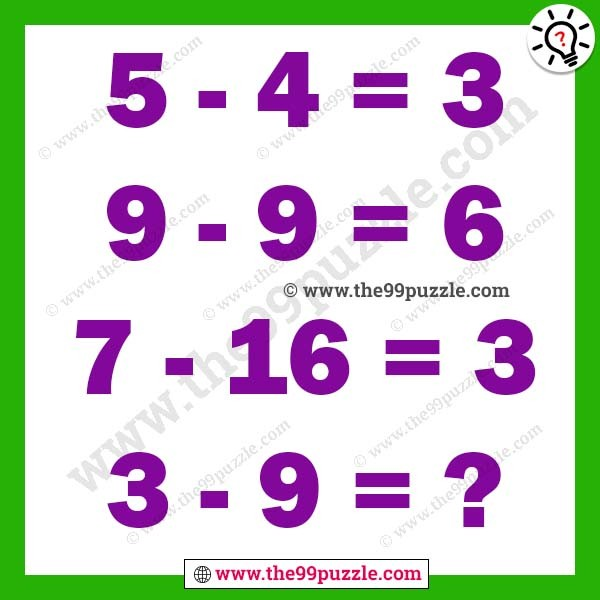# Tough math logical brain teaser with answer – Puzz172

Tough math logical brain teaser with answer. This is a very simple and interesting maths logic problem that will challenge your brain. Solving this maths logic puzzle will help you to improve your mathematical and logical reasoning skills. If you are intelligent people you can easily solve these puzzles?

5-4=3

9-9=6

7-16=3

3-9=?###### Explanation:

5-4 = 5-√4 = 5-2 = 3

9-9 = 9-√9 = 9-3 = 6

7-16 = 7-√16 = 7-4 = 3

3-9 = 3-√9 = 3-3 = 0